﻿ Generate a Text / ASCII Pyramid

Color

Background

Done

# Generate a Text / ASCII Pyramid

by kirupa   |   filed under Coding Exercises

From the earliest days of computers with screens, people have been creating art using nothing more than letters. A class of popular games from the 80's and 90's are fully immersed in this aesthetic. In this exercise, we are going to pay homage to that era by printing a pyramid to our console that looks as follows:This pyramid can have varying heights ranging from 1 to something much larger...like 25: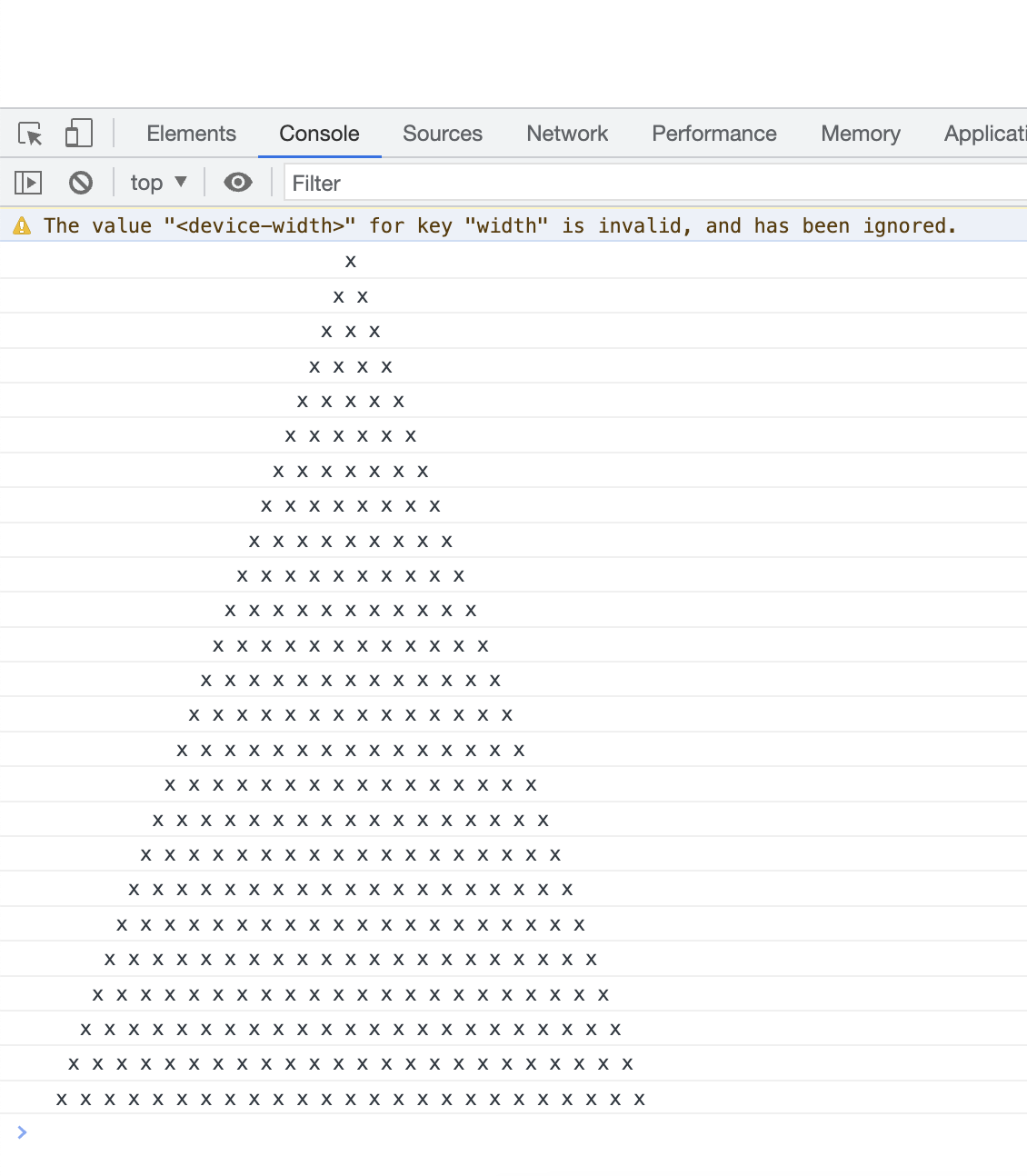One crucial detail to call out is that each row in this pyramid is properly center aligned. Pulling off that centering in the DOM is easier thanks to CSS. Our goal here is to use the browser's console, so that will require some extra creative thinking.

Onwards!

#### Hint: Some Learning Resources and Getting Help

The following tutorials may provide some helpful tips and techniques to help you with this exercise: Console Logging Basics,Looping in JavaScript, Strings, String.repeat()

We want to make this a fun learning activity. If you are stuck and need help, please ask on the forums. Please explain your problem in detail and one of the many helpful forum members will help you out.

Once you have completed this exercise, you have earned the tremendous bragworthy privilege of adding the following badge to your collection:To claim it, head to the forums and respond in the ASCII/Text Pyramid topic. Be sure to include a link to your solution or insert a copy of your HTML/CSS/JS in the body of the message. Once you have created your topic, Kirupa will give you a virtual high-five and ensure this badge is added to your list of assigned badges.

## One Possible Solution

As with all coding exercises, there are a billion ways to solve them. Below, we'll walk through one approach:

The first thing to do is take a step back and look at the problem at hand. If we had to visualize what it is we are creating without thinking about the console or any other extra visual noise, it would look like the following: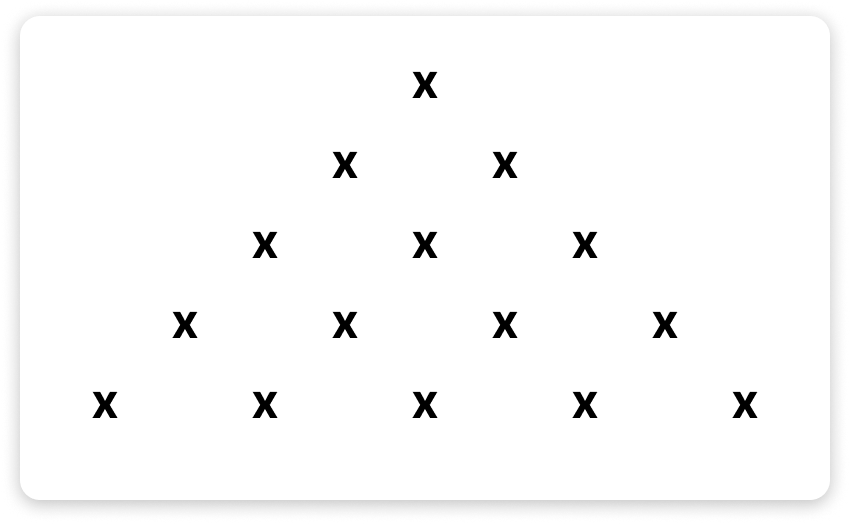We have some x characters arranged to look like a pyramid. These characters are arranged in a way to ensure that each row is perfectly centered, something that we will have to think about as well. Whenever we see a spatial arrangement of items that we need to re-create, our first reaction should be to map these items to a grid.

Extending that thought, we can think about each x character as being part of a grid made up of rows and columns: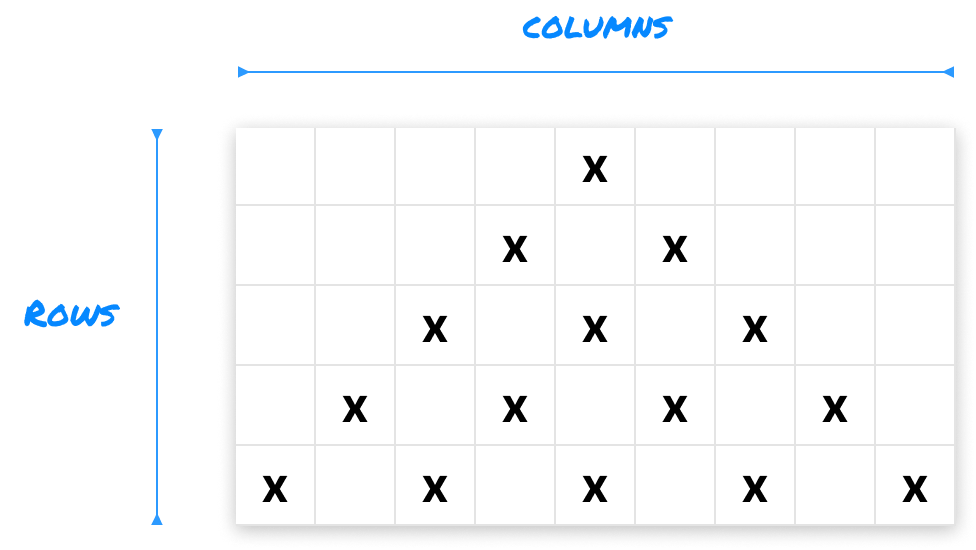When we add the grid and column representation, we have a bit more clarity on what we are trying to do. Each cell either stores an x character or a space character. These space characters are important in ensuring our pyramid looks balanced and centered!

To go deeper in helping both us and (eventually) our code make sense of this visual arrangement, we are going to give each row and column a number starting with 0: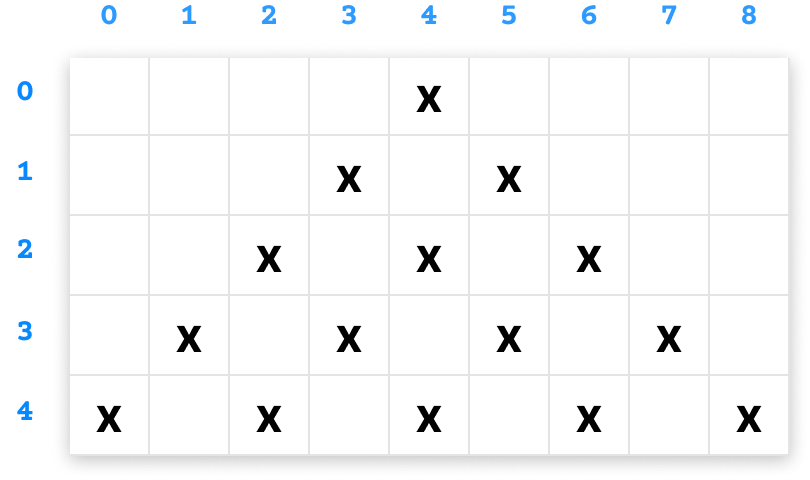We are starting with 0 because so many things in JavaScript (such as string character indexes, array indexes, etc.) all start their counting from 0 and then move on from there. We are in a good spot now. We simplified our original problem into something we can generalize as a pattern of rows and columns. It's time to go to the next stage!

Ignoring our computers for a moment, if we had to explain to another human being how to create a pyramid like this, how would we do so using English? It could look as follows for a pyramid that has a depth of 5:

1. At Row 0, we have an x at column position 4 (which is 1 less than the depth value of 5)
2. At Row 1, we have two x characters: one x is at column position 3 and another x is at column position 5
3. At Row 2, we have three x characters: one x at column position 2, one x at column position 4, and another x at column position 5

Stopping for this moment, let's make some assumptions about a pattern we can potentially describe:

1. The number of x characters in each row is 1 more than the row number. For example, Row 0 has 1 x character. Row 1 has 2 x characters.
2. The number of leading spaces before the first x character appears is 1 less than the depth. At Row 0 for our pyramid of depth 5, we have 4 space characters before the x appears at column position 4.

The second point above is an interesting one, for we are introducing one more way of visually thinking about our problem. We are making a distinction between the leading spaces that appear before the first x character and the spaces that appear between each x character. To simplify this, let us represent a leading space as an o, and let us represent an x character as xo to capture the space that follows them: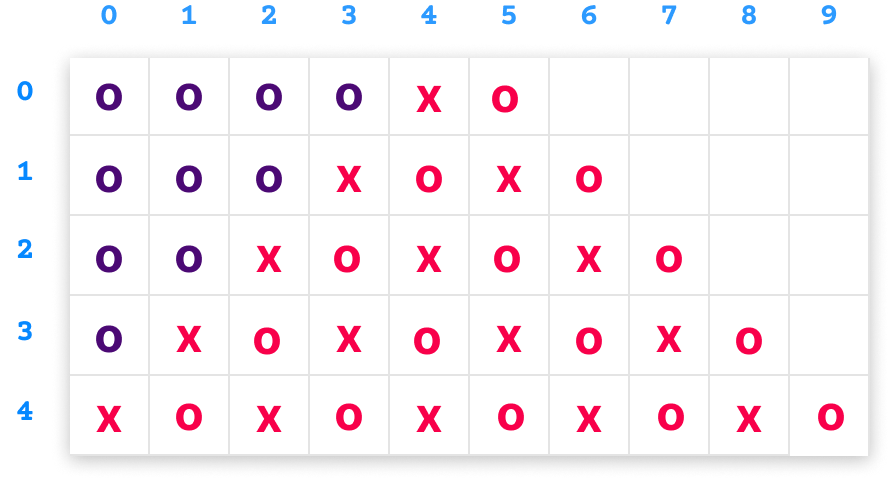Here we can now see that a leading space is represented as a purple o, and the x character is represented as an xo. Let's take the assumptions we described earlier and start translating the output into code.

The first thing we want to do is print out the leading spaces for each row. We are going to pull that off by using a for loop and the repeat method that allows us to repeat a string a specified number of times:

``````<!DOCTYPE html>
<html lang="en">

<meta charset="UTF-8">
<meta http-equiv="X-UA-Compatible" content="IE=edge">
<meta name="viewport" content="width=device-width, initial-scale=1.0">
<title>Pyramid Tutorial</title>

<body>
<script>
let x = "xo";
let space = "o";

let depth = 5;

for (let i = 0; i < depth; i++) {
console.log(space.repeat(depth - i));
}
</script>
</body>

</html>``````

The x and space variables store the two character variants we are dealing with. The for loop starts at 0 and goes until the depth value. This mimics the number of rows in our pyramid where, for a pyramid of depth 5, we start at 0 and go all the way to 4.

At each loop iteration, we print the number of leading space characters by using the repeat method and repeating the character by depth minus the value of i:

``````for (let i = 0; i < depth; i++) {
console.log(space.repeat(depth - i));
}``````

We print the output of each iteration to our console, and this is what our ouput looks like right now: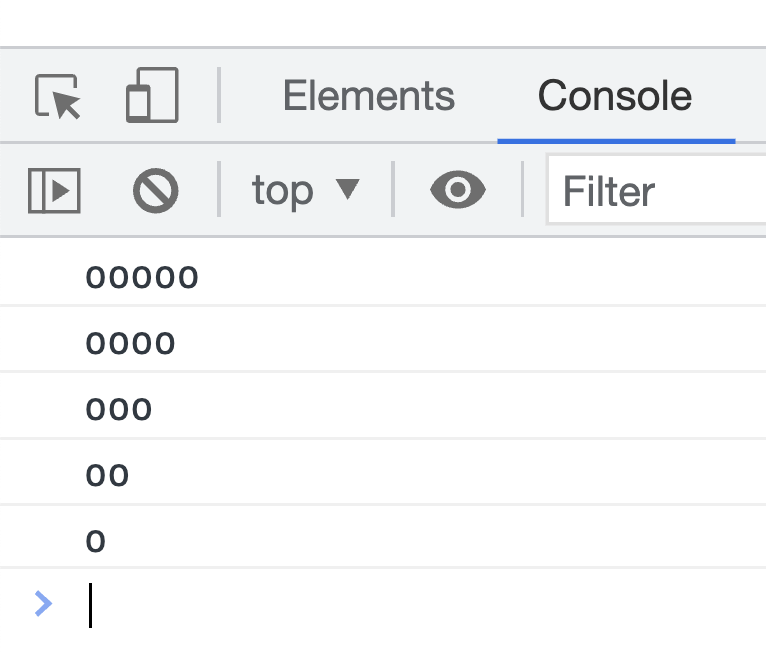Pay close attention to the output. Something is off right? At Row 0, we should see 4 leading spaces. What we are seeing is 5 leading spaces. The reason is that there is flaw in one of our assumptions. We said that we repeat each leading space character by the current depth minus 1. What we should be doing is accounting for the x character that appears in place of the last leading space. This means our repeat count should really be as follows:

``````for (let i = 0; i < depth; i++) {
console.log(space.repeat(depth - i - 1));
}``````

We need to take one more count away to ensure we have the right number of leading spaces. When we look at the output at this time, this will look more accurate: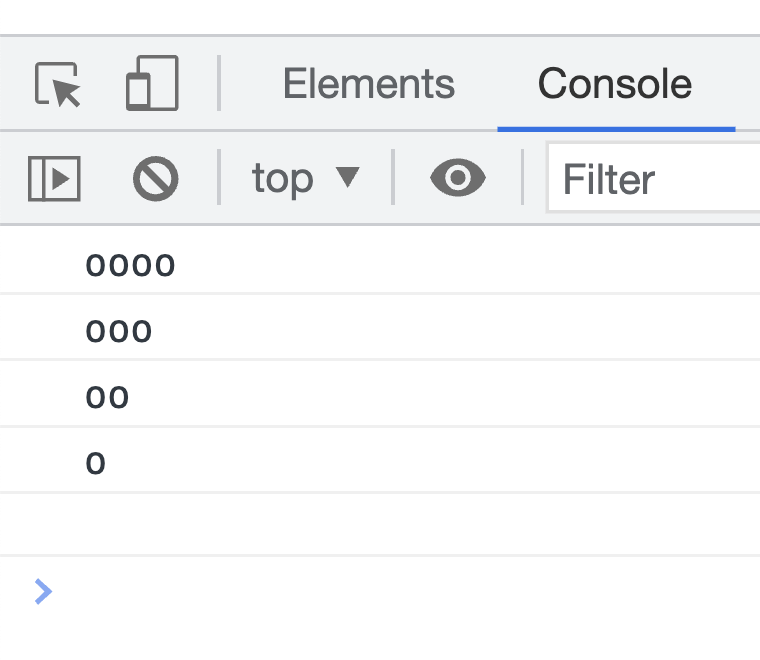As you and I do more exercises like this, there will be many moments where we need to double-check our assumptions and make adjustments based on what we learn along the way. That's what makes all of this a fun learning experience that mimics what programming is often really like...a lot of trial and error!

Now that we have our leading space sorted out, let's add our logic for displaying our x characters. From one of our earlier assumption, the number of x characters is 1 more than the row number. The code for that would look as follows:

``````for (let i = 0; i < depth; i++) {
let leading_spaces = space.repeat(depth - i - 1);
let x_characters = x.repeat(i + 1);

}``````

We made our code a bit more readable by creating a dedicated leading_spaces variable to store our leading spaces characters, and the code for representing the number of x characters is represented by the x_characters variable. Notice that we directly translated our English description into code where we repeat the x characters by our row count (represented by i) plus 1.

If we look at our console now, what we see will look as follows: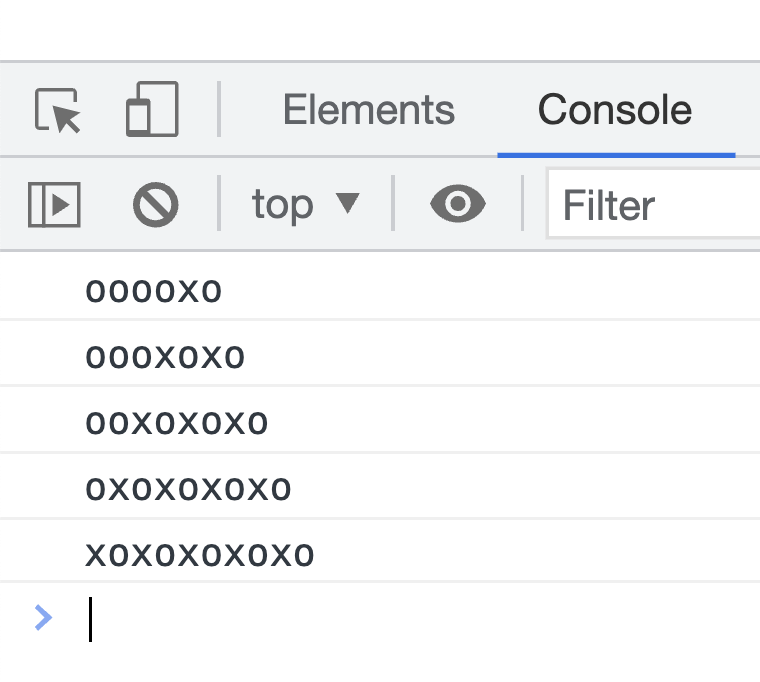The arrangement of leading spaces to x characters looks accurate. What we can do as a last step is replace our space character 0 with an actual space. We can do that by changing the value of our x and space variables:

``````let x = "x ";
let space = " ";

let depth = 5;

for (let i = 0; i < depth; i++) {
let leading_spaces = space.repeat(depth - i - 1);
let x_characters = x.repeat(i + 1);

}``````

When replace the o with an empty space character, our output in the console looks more like the problem we originally started off with wanting to solve:Just for kicks, if we increase our depth value to 25 to represent a much larger pyramid, our pyramid will look as follows:That looks pretty awesome, right? Now, there is a bit more we can do to make this output. If you have some suggestions, alternate ways of solving this, or just have some questions, please do share this in the How to Print this Pyramid Pattern thread!